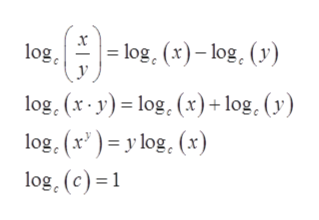# Write the logarithm as a sum or difference of logarithms. Simplify each term as much as possible. Assume that the variables represent positive real numbers. Log3 (1/9 a3 b2 ) =

Question
6 views

Write the logarithm as a sum or difference of logarithms. Simplify each term as much as possible. Assume that the variables represent positive real numbers.

Log3 (1/9 a3 b2 ) =

check_circle

Step 1

Given, logarithm expression is

Step 2

Formula nee...help_outlineImage Transcriptionclose= log. (x)– log. (y) log. log, (x - y) = log, (x)+ log. (v) log, (x' ) = y log, (x) log, (c) =1 fullscreen

### Want to see the full answer?

See Solution

#### Want to see this answer and more?

Solutions are written by subject experts who are available 24/7. Questions are typically answered within 1 hour.*

See Solution
*Response times may vary by subject and question.
Tagged in

### Algebra